# Classroom

There are eighty more girls in the class than boys. Boys are 40 percent and girls are 60 percent. How many are boys and how many girls?

Correct result:

a =  240
b =  160

#### Solution:

a = 80+b
a = 0.6 (a+b)

a = 80+b
a = 0.6•(a+b)

a-b = 80
0.4a-0.6b = 0

a = 240
b = 160

Our linear equations calculator calculates it.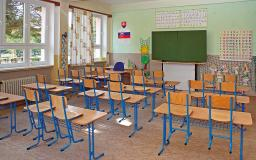We would be pleased if you find an error in the word problem, spelling mistakes, or inaccuracies and send it to us. Thank you!Tips to related online calculators
Do you have a linear equation or system of equations and looking for its solution? Or do you have quadratic equation?

## Next similar math problems:

• PupilsThere are 32 pupils in the classroom, and girls are two-thirds more than boys. a) How many percents are more girls than boys? Round the result to a whole percentage. b) How many are boys in the class? c) Find the ratio of boys and girls in the class. Writ
• In the classroomThere are 30 boys and a few girls in the class. In the six months, 28 boys and all girls benefited, which was 95% of all pupils. How many pupils are there in the classroom?
• Cross-country competition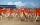Cross-country competition was attended by 76% of pupils of class 9.B. Class consis of 16 boys, two of whom did not run and 4 girls did not run. How many girls go to class 9.B?
• The farmer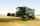The farmer harvested 840 tons of grain in 2006, which was 44% less than in 2005, and one-fifth more than in 2004. How many tons of grain harvested in years 2005 and 2004?
• EmployeesThere are 1116 people working in three factory halls. In the first one, there are 18% more than the third, and 60 persons more than the second. How many employees work in individual halls?
• Two math problems1) The sum of twice a number and -6 is nine more than the opposite of that number. Find the number. 2) A collection of 27 coins, all nickels, and dimes, is worth \$2.10. How many of each coin are there? The dime, in United States usage, is a ten-cent coin.
• 3 days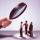Worker checked 2,950 products in 3 days. Second day checked 25% more than the first day. The third day 15% more products than the second day. How many products he checked in each day.
• Two workers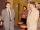Two workers make 138 parts/items. The first one produces 30% more than the second. How many items will each produce?
• Three piles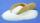100 kg of sugar we divide into three piles. The first pile is small. If we added to second 2 kg of sugar it would have 25% more sugar than the first pile. If we added to third pile 3 kg of sugar it would have 20% more sugar than the 2nd pile. How many kil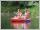Students of canoeists trip traveled in three days 102 km. Second day traveled 15% more than first day and at the third day 9 km over the second day. How many kilometers traveled each day?
• Company and employeesThere are 370 employees in the company - women are 15% less than men. How many men work in the company?
• Ski class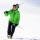Class attend 30 boys and some number of girls. Ski training was attended by 28 boys and all the girls, which was 95% of all students. How many girls attend this class? How many percent is that?
• Chickens and rabbitsIn the yard were chickens and rabbits. Together they had 27 heads and 86 legs. How many chickens and how many rabbits were in the yard?
• Crowns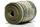1180 crowns is divided into three people that A got 20% less than B and C by 15% more than B. How many got A,B and C?
• School year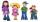At the end of the school year has awarded 20% of the 250 children who attend school. Awat got 18% boys and 23% of girls. Determine how many boys and how many girls attend school.
• PropertyThe length of the rectangle-shaped property is 8 meters less than three times of the width. If we increase the width 5% of a length and lendth reduce by 14% of the width it will increase the property perimeter by 13 meters. How much will the property cost
• Tickets1260 tickets sold. On the first day, 80% was sold on the second day was sold. How many tickets were sold first and how much the next day?